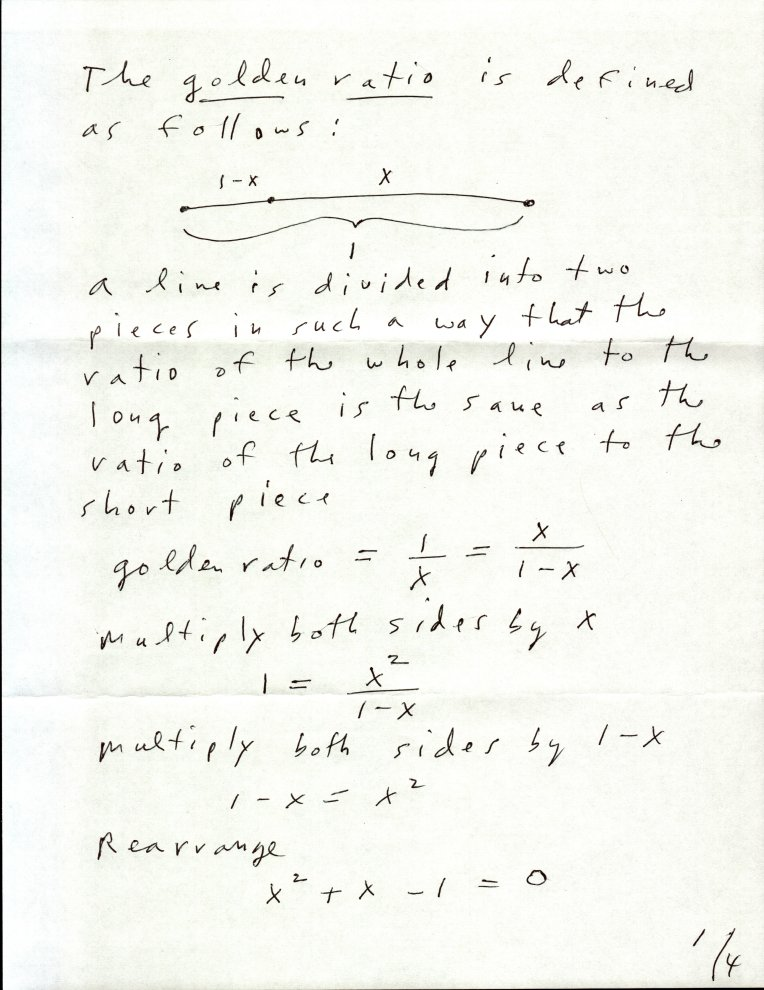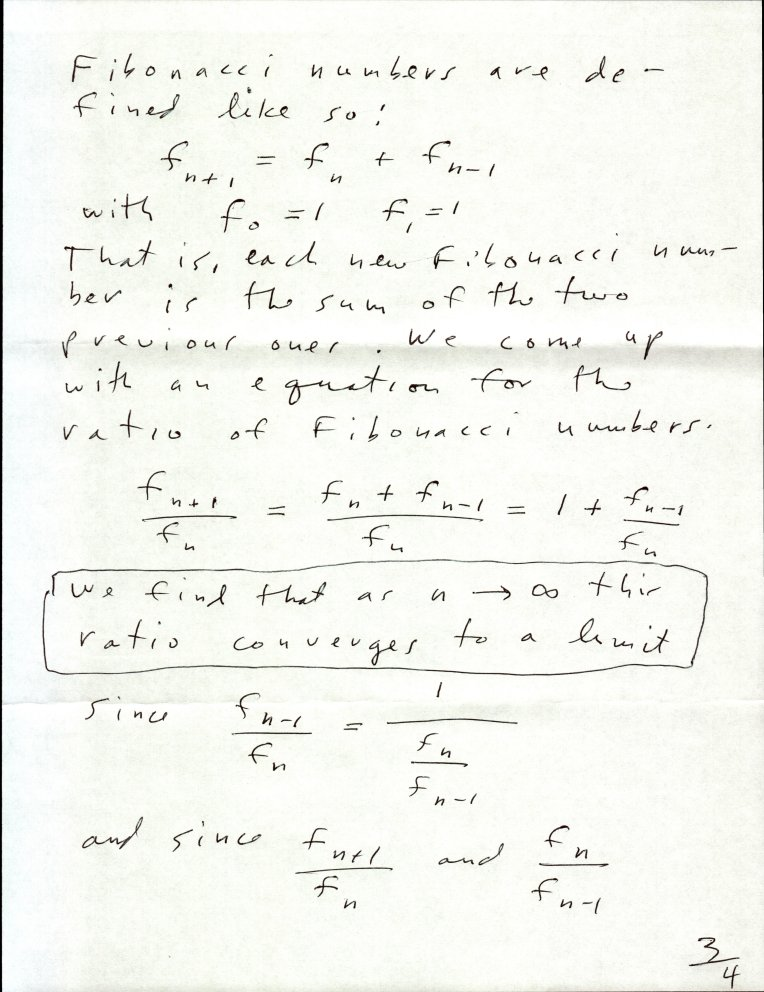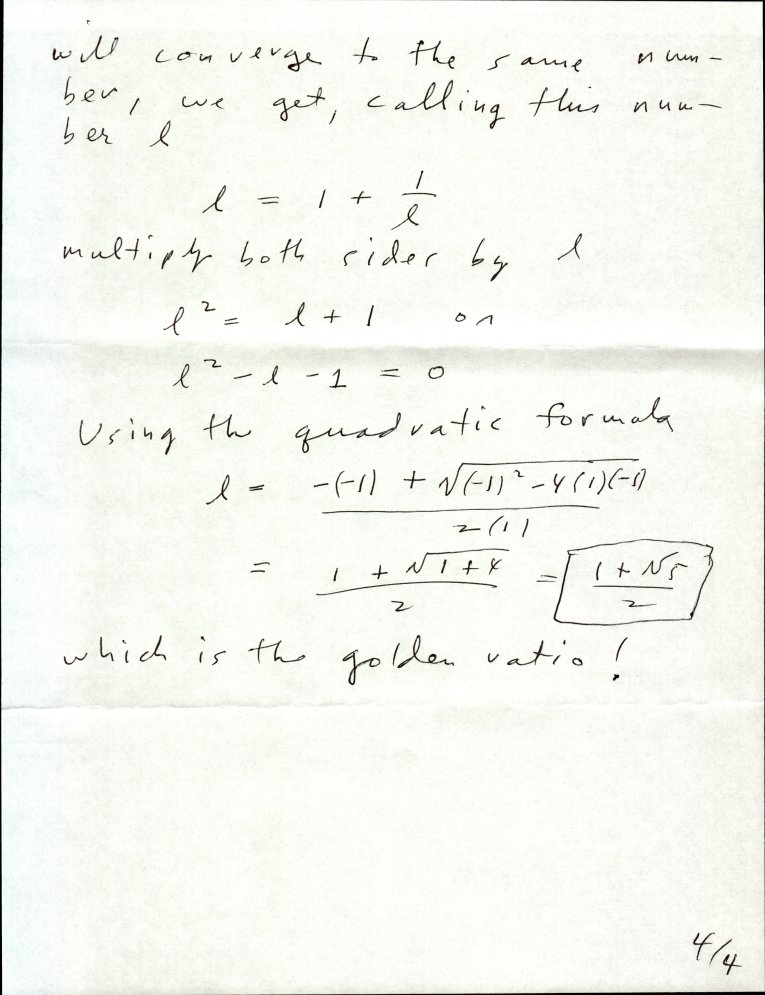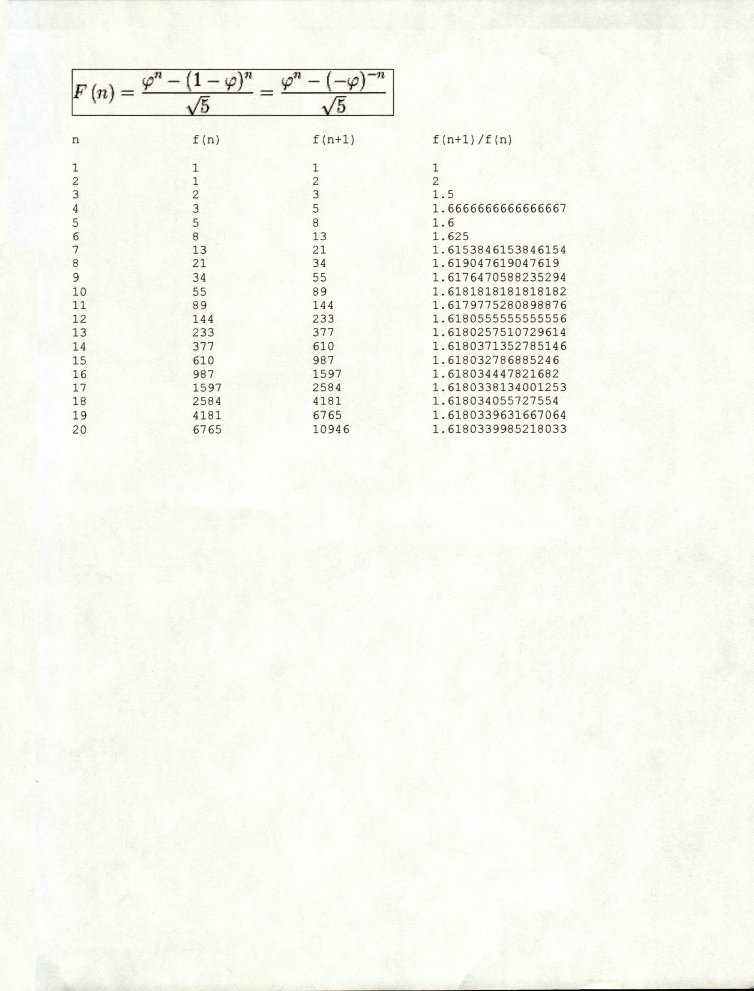The Golden RatioA closed-form expression for the n-th Fibonacci number (discovered by Jacques Philippe Marie Binet, though it was already known by Abraham de Moivre and Daniel Bernoulli) is shown below. φ is the golden ratio.A tiny-C program which uses this formula via a "plugin" athttp://primepuzzle.com/not.just.tiny.c/pifib.c is athttp://primepuzzle.com/not.just.tiny.c/fibpi.tc ```D:\my-git-repo>tc fibpi.tc *** TINY-C VERSION 1.0, COPYRIGHT 1977, T A GIBSON *** This C version copyright 2017, T A Gibson fibpi.tc - lrb - 6/25/21 enter a number < 40 39 fib(40) = 102334155 fib(39) = 63245986 ratio of these two Fibonacci numbers is 1.61803398874989473640 done D:\my-git-repo>tc fibpi.tc *** TINY-C VERSION 1.0, COPYRIGHT 1977, T A GIBSON *** This C version copyright 2017, T A Gibson fibpi.tc - lrb - 6/25/21 enter a number < 40 20 fib(21) = 10946 fib(20) = 6765 ratio of these two Fibonacci numbers is 1.61803399852180339985 done D:\my-git-repo> For an interesting and creative discussion of how this ratio appears in sunflowers Chip Bradley posted the following. http://primepuzzle.com/not.just.tiny.c/sunflower.whorls.html ```